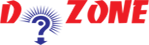﻿ Python Program to Convert Kilometers to Miles## Python Program to Convert Kilometers to Miles

So, here in this program you will learn how to convert Kilomenters to Miles by using Python programming. Hers is the formula to convert Kilometers to Miles

``````
kilometers * conv_fac = miles
```
```
``````
Input
kilometers = 8.5

# To take kilometers from the user, uncomment the code below
# kilometers = float(input("Enter value in kilometers"))

# conversion factor
conv_fac = 0.621371

# calculate miles
miles = kilometers * conv_fac
print('%0.3f kilometers is equal to %0.3f miles' %(kilometers,miles))
Output
8.500 kilometers is equal to 5.282 miles
```
```

Conclusion: To test the program simply change the values in the program. Also, if you want to convert miles to kilometers use the given formula and run it.

``````
kilometers = miles / conv_fac
```
```

## Other Python Programs

PythonTraining, 258-FF , Katewa Nagar, New Sanganer Rd, Jaipur, 302019